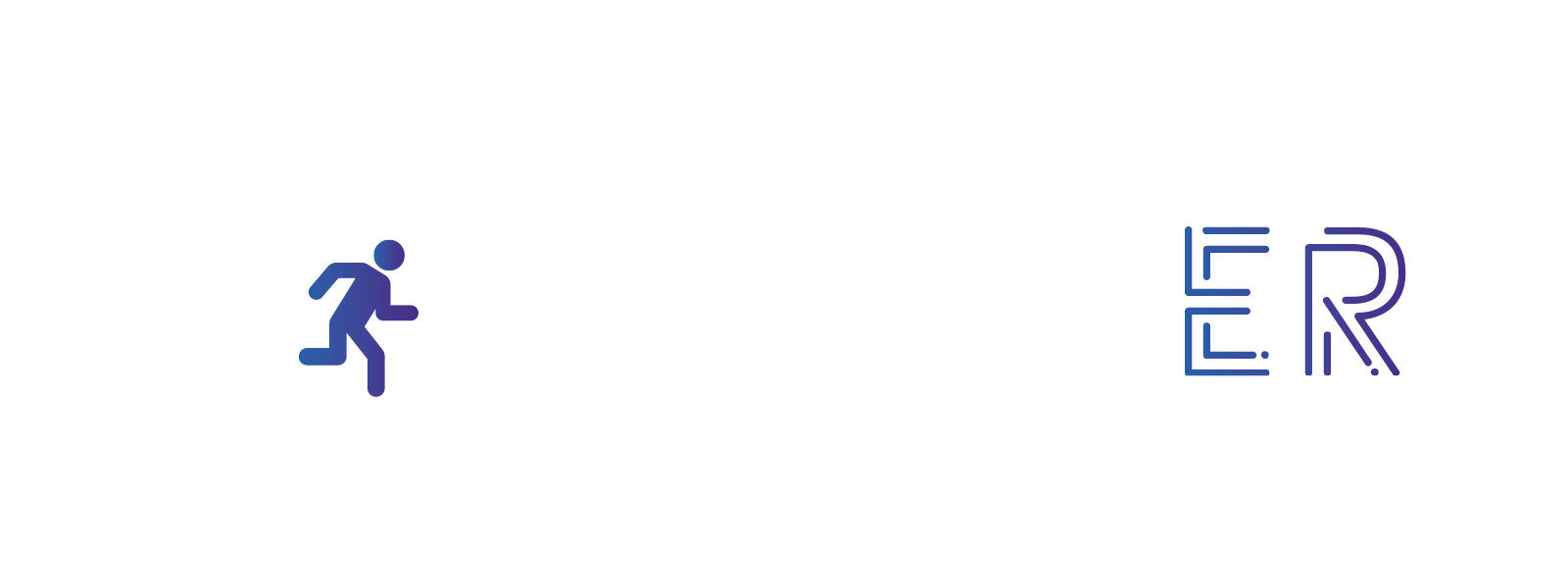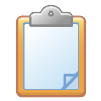Students must correctly answer the four ABCD questions. Correct answers will give them a four-digit code.
Does it require an electronic device? No

1. Students receive a worksheet with questions. Each question has four answer suggestions.

2. The answers are not marked with the letters ABCD. Numbers were used instead of letters.

 Example version simple: Example version advanced – letters to alphabetic numbers (a=1, z=26) instead of letter A, number 9, instead of letter B, number 6, instead of letter C, number 2, instead of letter D, number 3. If we need number 9 – letter I If we need number 6 – letter F If we need number 2 – letter B If we need number 3 – letter C

3. Simple version: after selecting the answer, students receive four digits – this is the code.

Advanced version: after selecting the answer, students receive four letter code. Each letter should be converted to a number. The number of the appropriate letter is its order in the NAVO alphabet:

A=1, B=2, C=3, D=4, E=5, F=7, G=8, H=9

*Attention! in this case there is no 0

4. The code opens the padlock with the next puzzle / element needed, etc.

Find it here